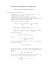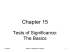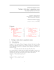# Inferential Statistics t and ScWk 242 – Session 9 Slides

## Transcription

Inferential Statistics t and ScWk 242 – Session 9 Slides
```Inferential Statistics
and t - tests
ScWk 242 – Session 9 Slides
Inferential Statistics
 Inferential statistics are used to test hypotheses
about the relationship between the independent
and the dependent variables.
 Inferential statistics allow you to test your
hypothesis
 When you get a statistically significant result
using inferential statistics, you can say that it is
unlikely (in social sciences this is 5%) that the
relationship between variables is due to chance.
3
• Statistics NEVER prove anything, instead, they
indicate a relationship within a given probability
of error.
• An association does not necessarily indicate a
sure cause effect relationship.
• Statistics can always be wrong, however, there
are things that researchers can do to improve the
likelihood that the statistical analysis is correctly
identifying a relationship between variables.
4
Probability Theory
 Probability theory: Allows us to calculate the exact probability that
chance was the real reason for the relationship.
 Probability theory allows us to produce test statistics (using
mathematical formulas)
 A test statistic is a number that is used to decide whether to accept or
reject the null hypothesis.
 The most common statistical tests include:
•
•
•
•
•
Chi-square
T-test
ANOVA
Correlation
Linear Regression
5
Normal Distributions
• All test statistics that use a continuous dependent
variable can be plotted on the normal distribution (chisquare, for example, uses the chi-square distribution).
• A normal distribution is a theoretical bell shaped curve:
6
Significance – Rejection Regions
• If the test statistic produced by the statistical test (using a
mathematical formula) falls within a specified rejection region on the
normal distribution, then we can conclude that the relationship
between the independent and dependent variables is unlikely to be
due to chance. (rejection = rejection of the NULL hypothesis)
• The rejection region is determined by the researcher prior to
conducting the statistical test and is called the alpha level.
7
Two-Tailed Significance Tests
• Two-tailed statistical tests (most common) split the rejection
region between the tails of the normal distribution so that
each tail contains 2.5% of the distribution for a total of 5%.
• Two-tailed tests test non-directional hypotheses
• Example:
• It is hypothesized that there is a relationship between participation in
Independent Living Programs while in foster care (the independent
variable) and having been taught budgeting skills while in foster care (the
dependent variable)
• We are not specifying whether the ILP group is more or less likely to have
been taught budgeting skills while in foster care
• We are just saying that there is a difference in the dependent variable
(budgeting skills) between the two groups (ILP vs. no ILP)
• Researchers usually choose two-tailed tests to allow for the possibility
that the IV affects the DV in the opposite direction as expected
8
One-Tailed Tests
One-tailed tests test directional hypotheses
Example:
• It is hypothesized that youth who participated in
Independent Living Programs while in foster care (the
independent variable) will have a greater likelihood of
having been taught budgeting skills while in foster care (the
dependent variable)
• We are specifying the expectation that ILP youth will be
more likely to have been taught budgeting skills while in
foster care than non-ILP youth
• The possible risk with one-tailed tests of directional
hypotheses is that if ILP youth have fewer budgeting skills,
the test won’t pick it up and we would have missed a
significant finding (in an unexpected direction).
9
p Values
• Each test statistic has a p value (a probability value)
associated with it.
• When you plot a test statistic on the normal
distribution, the location of the test statistic on the
normal distribution is associated with a p value, or a
probability.
• If the p value produced by the test statistic is within the
rejection region on the normal distribution, then you
reject the null hypothesis and conclude that there is a
relationship between the independent and the
dependent variables. This shows statistical significance.
10
t-test Statistic
• The t statistic allows researchers to use sample data to test
hypotheses about an unknown population mean.
• The t statistic is mostly used when a researcher wants to
determine whether or not a treatment intervention causes
a significant change from a population or untreated mean.
• The goal for a hypothesis test is to evaluate the significance
of the observed discrepancy between a sample mean and
the population mean.
• Therefore, the t statistic requires that you use the sample
data to compute an estimated standard error of M.
• A large value for t (a large ratio) indicates that the obtained
difference between the data and the hypothesis is greater
than would be expected if the treatment has no effect.
11
Significance vs. Magnitude
• Degrees of Freedom (df) is computed by using n – 1
with larger sample sizes resulting in an increased
chance of finding significance.
• Because the significance of a treatment effect is
determined partially by the size of the effect and
partially by the size of the sample, you cannot
assume that a significant effect is also a large effect.
• Therefore, it is recommended that the measure of
effect size (differences of outcomes vs. expectations)
be computed along with the hypothesis test.
12
Interpreting Results
Key items to include in the
interpretation of results:
•Are the findings consistent, or
not, with previous research?
•Clinical relevance (different from
statistical significance)
•Limitations and Potential Errors
13
Happy Spring Break!
14
```

### Population vs. Sample - Ms. Tomas` Math Page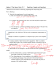### HYPOTHESIS TESTING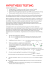### File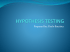### Chapter 9: Testing a Claim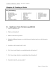### Document 6537334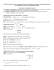### Hypothesis Testing Series (One Sample) STA2023-4a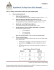### sonia cordero cardenas san andres university – la paz •jaime rojas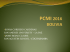### chapter 9 practice - faculty.piercecollege.edu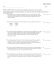### Chapter 11 and 12 Review 2015 ANSWERS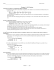### Review for the Final Exam (STA248)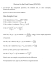### 155S8.5_3 Testing a Claim About a Mean: s Not Known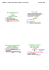### What is Hypothesis Testing? AP Statistics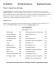### Math 210 - Exam 5 - Sample Exam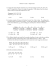### Stat 100 Sample Final Questions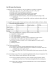### August 2012 EXAMINATIONS Solution – Part I (B)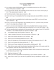### Math 116 - Final Review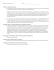### Final Review. More Problems. STAT 145 Problem 1. (formulation is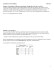### One-Sample Hypothesis Tests Review Problems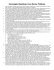### Review for Midterm II